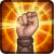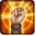# Instant d'héroïsmeConditions

## Used by

Triggered by using an item (1)

## Related effects, buffs and debuffs

• [0s] [not displayed]Effect #1

 Slot: Buff Duration: 0s Tick rate: does not tick # occurrences: 0
• On Apply

Only when the following conditions are met:

• If CASTER is one of these classes:
- 16140902893827567561

Perform the following actions:

• Modify Cooldown
- Is Percentage = (bool) true
- Tag = (string)
- Tags = (string)
- Ability Spec = (int) 16141144652231716538
- Amount Min = (float) 0
- Amount = (float) -1
• On Apply

Only when the following conditions are met:

• If CASTER is one of these classes:
- 16141010271067846579

Perform the following actions:

• Modify Cooldown
- Is Percentage = (bool) true
- Tag = (string)
- Tags = (string)
- Ability Spec = (int) 16140959936569960182
- Amount Min = (float) 0
- Amount = (float) -1
• On Apply

Only when the following conditions are met:

• If CASTER is one of these classes:
- 16141170711935532310

Perform the following actions:

• Modify Cooldown
- Is Percentage = (bool) true
- Tag = (string)
- Tags = (string)
- Ability Spec = (int) 16140973038486484207
- Amount Min = (float) 0
- Amount = (float) -1
• On Apply

Only when the following conditions are met:

• If CASTER is one of these classes:
- 16140943676484767978

Perform the following actions:

• Modify Cooldown
- Is Percentage = (bool) true
- Tag = (string)
- Tags = (string)
- Ability Spec = (int) 16140984008308729401
- Amount Min = (float) 0
- Amount = (float) -1
• On Apply

Only when the following conditions are met:

• If CASTER is one of these classes:
- 16141119516274073244

Perform the following actions:

• Modify Cooldown
- Is Percentage = (bool) true
- Tag = (string)
- Tags = (string)
- Ability Spec = (int) 16140995633548445924
- Amount Min = (float) 0
- Amount = (float) -1
• On Apply

Only when the following conditions are met:

• If CASTER is one of these classes:
- 16141179471541245792

Perform the following actions:

• Modify Cooldown
- Is Percentage = (bool) true
- Tag = (string)
- Tags = (string)
- Ability Spec = (int) 16141077268183510807
- Amount Min = (float) 0
- Amount = (float) -1
• On Apply

Only when the following conditions are met:

• If CASTER is one of these classes:
- 16140973599688231714

Perform the following actions:

• Modify Cooldown
- Is Percentage = (bool) true
- Tag = (string)
- Tags = (string)
- Ability Spec = (int) 16141163070451073612
- Amount Min = (float) 0
- Amount = (float) -1
• On Apply

Only when the following conditions are met:

• If CASTER is one of these classes:
- 16140912704077491401

Perform the following actions:

• Modify Cooldown
- Is Percentage = (bool) true
- Tag = (string)
- Tags = (string)
- Ability Spec = (int) 16140931428119054736
- Amount Min = (float) 0
- Amount = (float) -1
• When effect is applied
• When effect is refreshed

Perform the following actions:

• Play appearance epp . itm . premium . xp_boosts . class . major